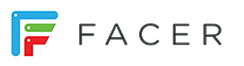# New Facer Editor UI

Hi there. This is just demo mockups of my vision to improving editor.
I use editor every day and this is only my minds.
If you like it or no plese let me know…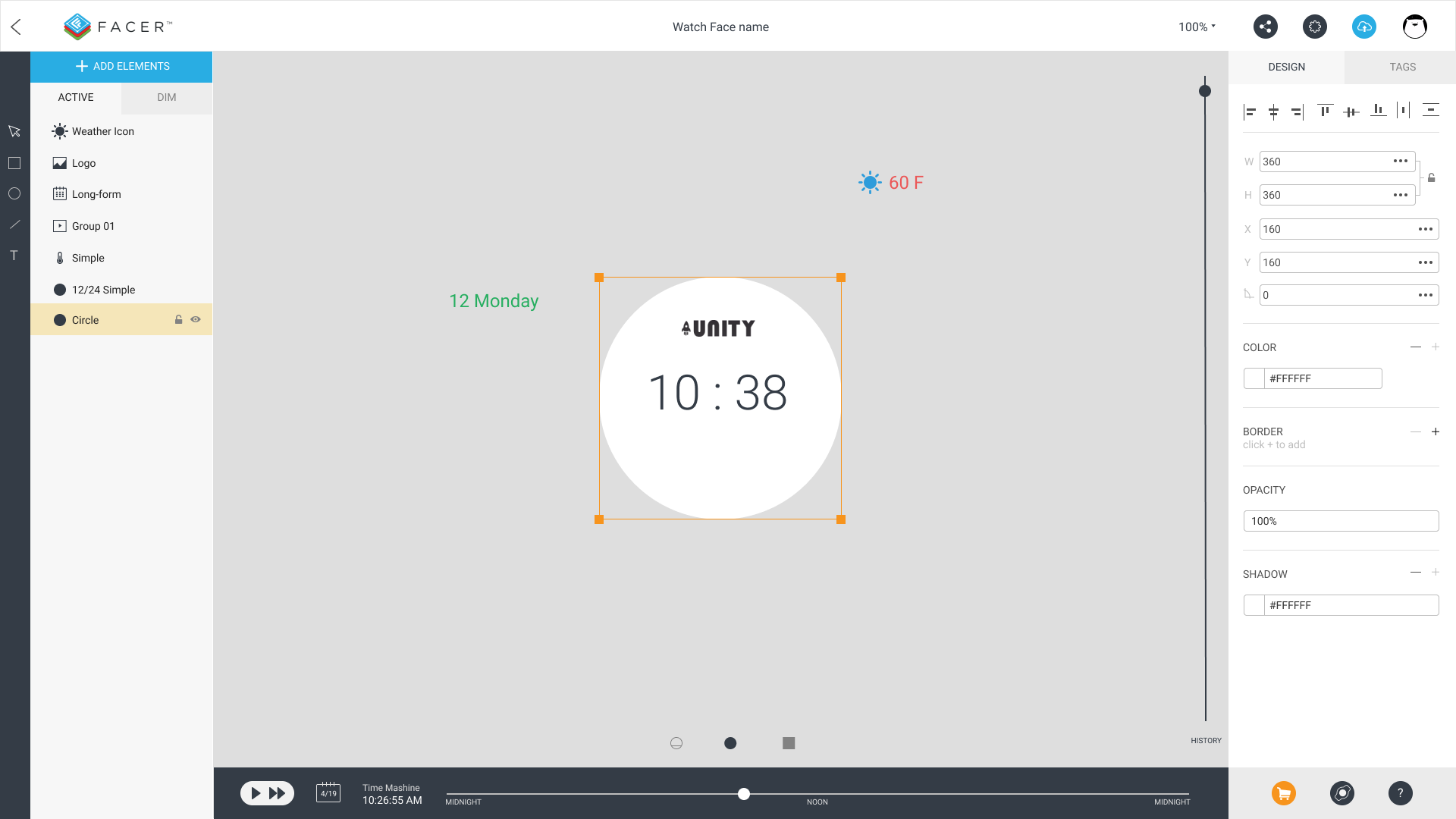1. Added tools pannel on the left.

2. Icons Lock/Unlock and save/unlock appears by Hover or select layer.
Layers can be duplicated (ctrl+j)

3.There is The Properties panel on the right. Always visible.
If any element is selected now data appears on the Properties panel. There are added elements alignment tools.

1. If Elements aren’t on an art board they are visible on Canvas. You can select elements without using layers. Art board icons (visibility mode selector) are above on Time Machine panel but I’m not sure if it’s good.

2. There is History panel on the right. It’s a scroll-bar. Pull it down to undo - up to redo.

3. There is zoom indicator (100%) on Top-bar panel.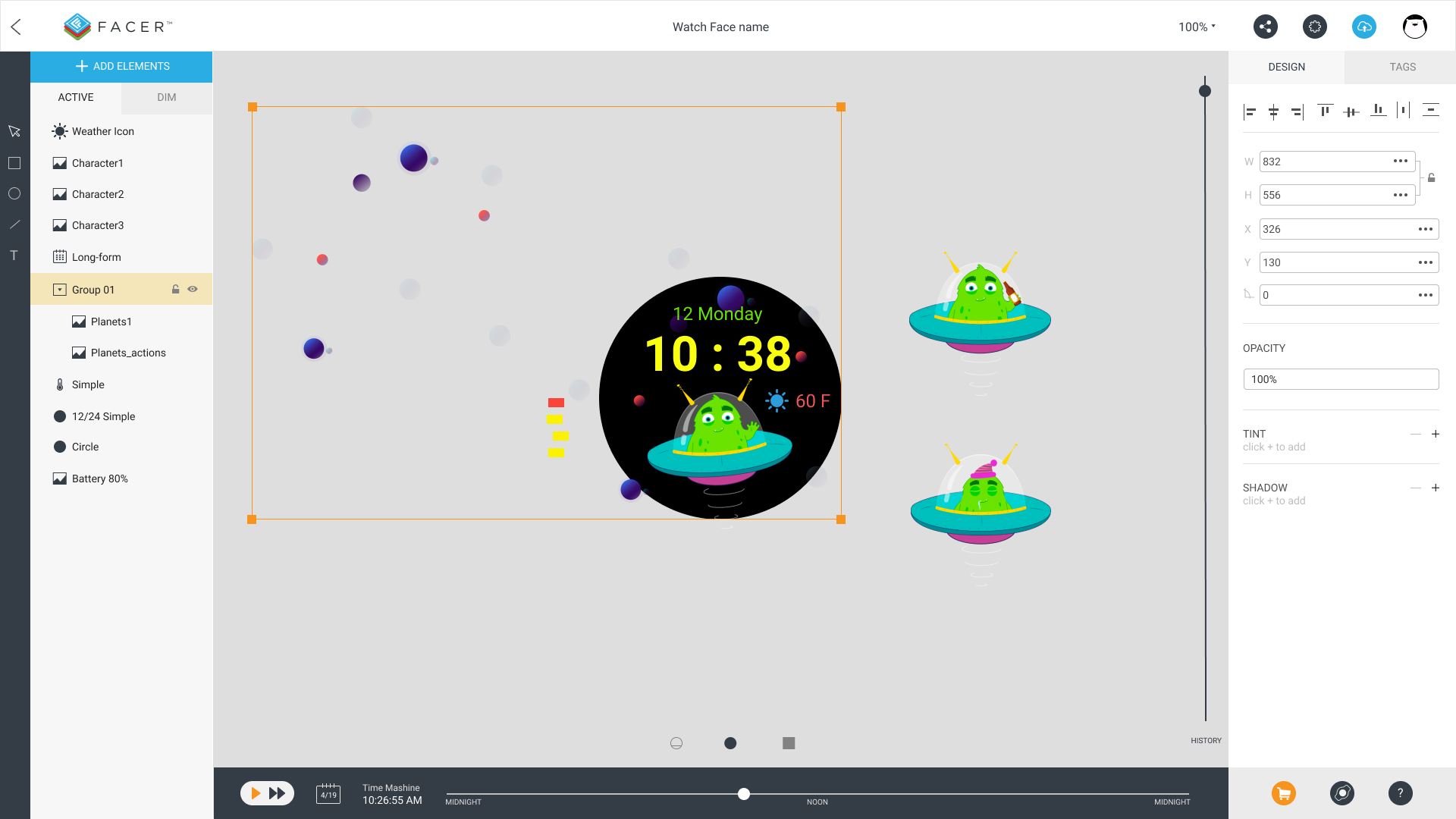1. All elements can be group (ctrl+g).
Common data of the group appeared on Properties panel.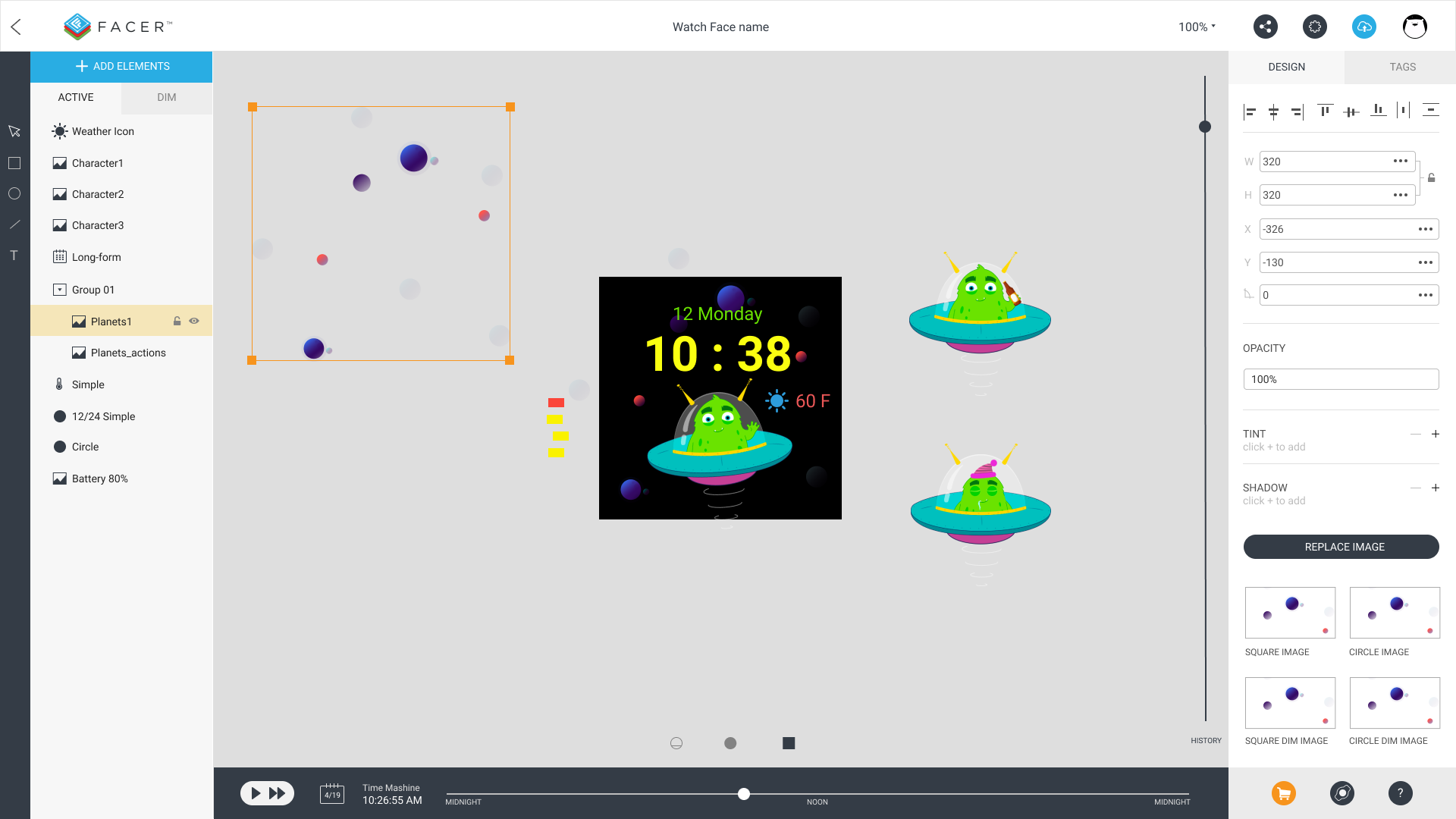1. Squared Artboard.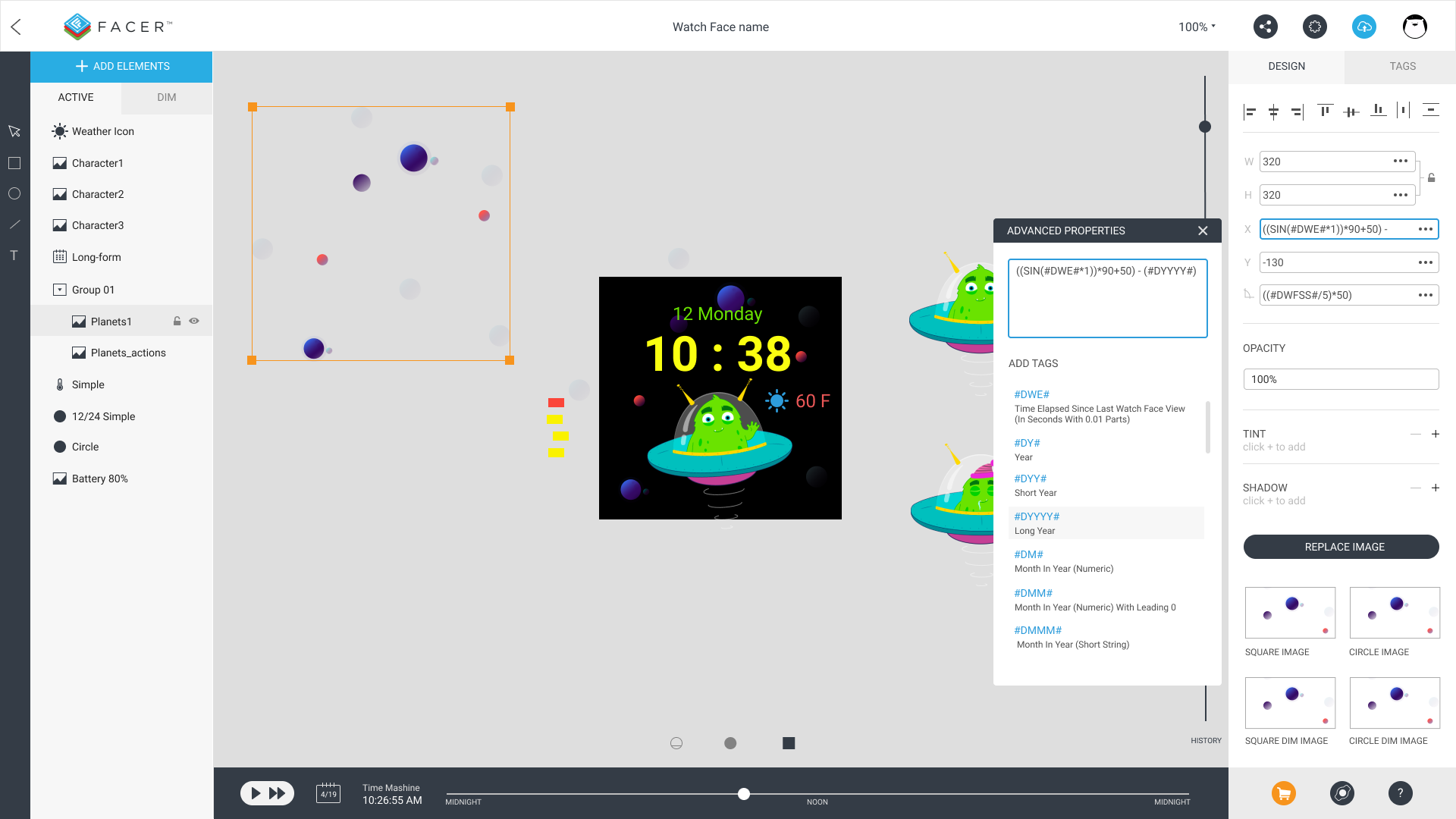1. Use More icon(…) to show Pop-over panel. It has additional elements properties/features.
Code input increased for better Tags coding.

2. All tags are in The Tags tab.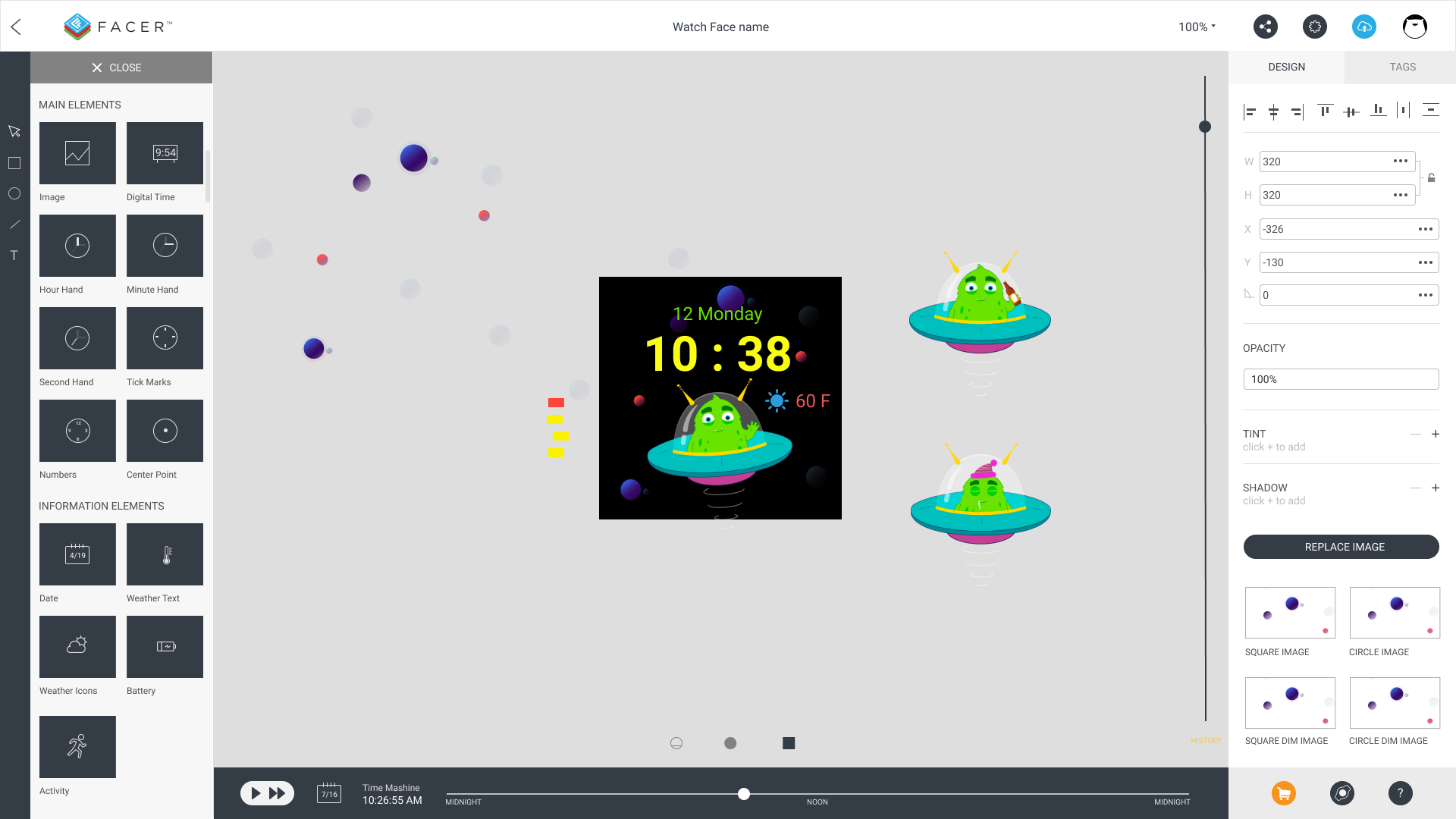1. Elements library opens by pushing the same button Add Elements.
It has 100% height for better use.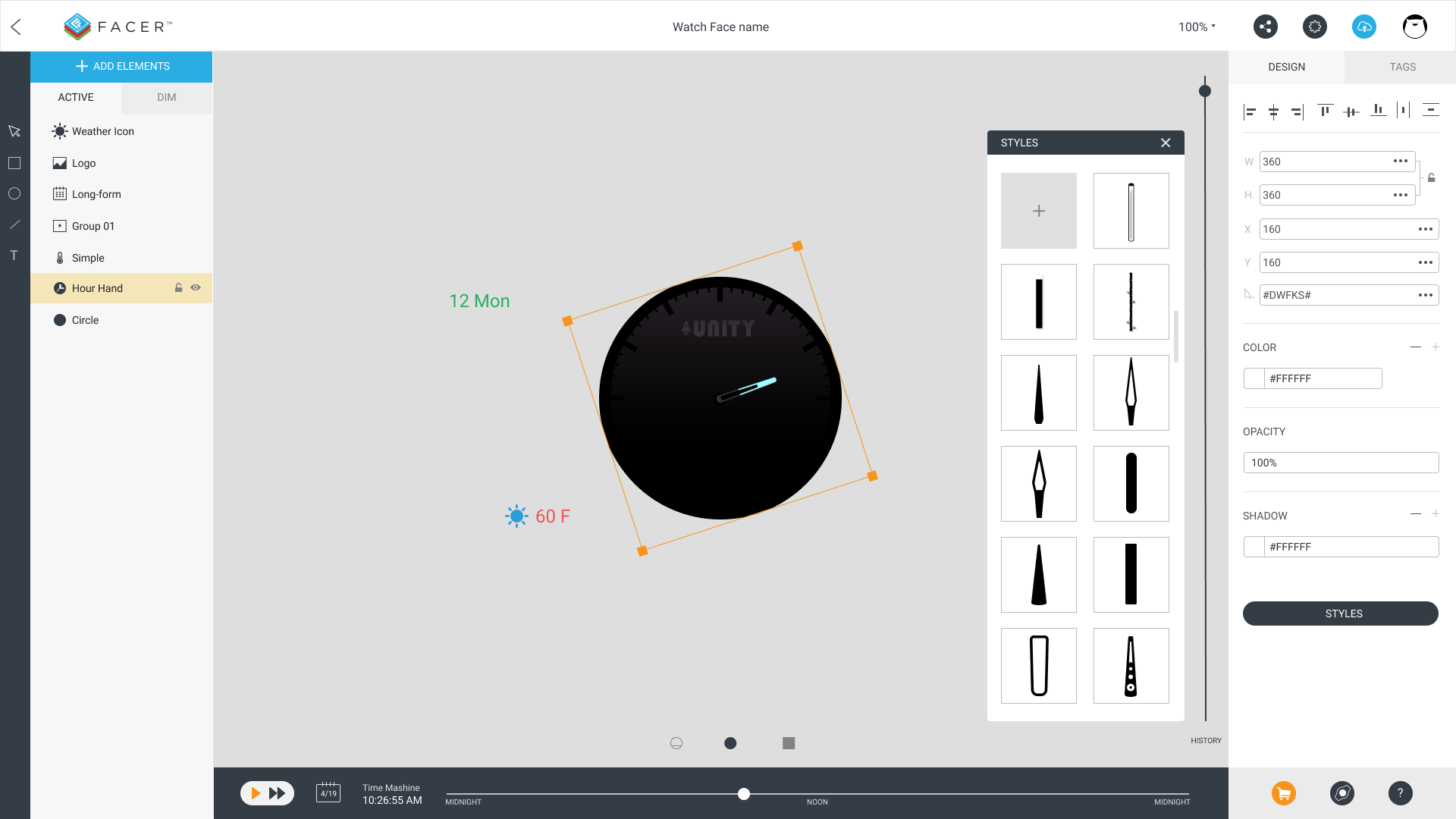1. If added element has additional parameter it will appear immediately in the special pop-over.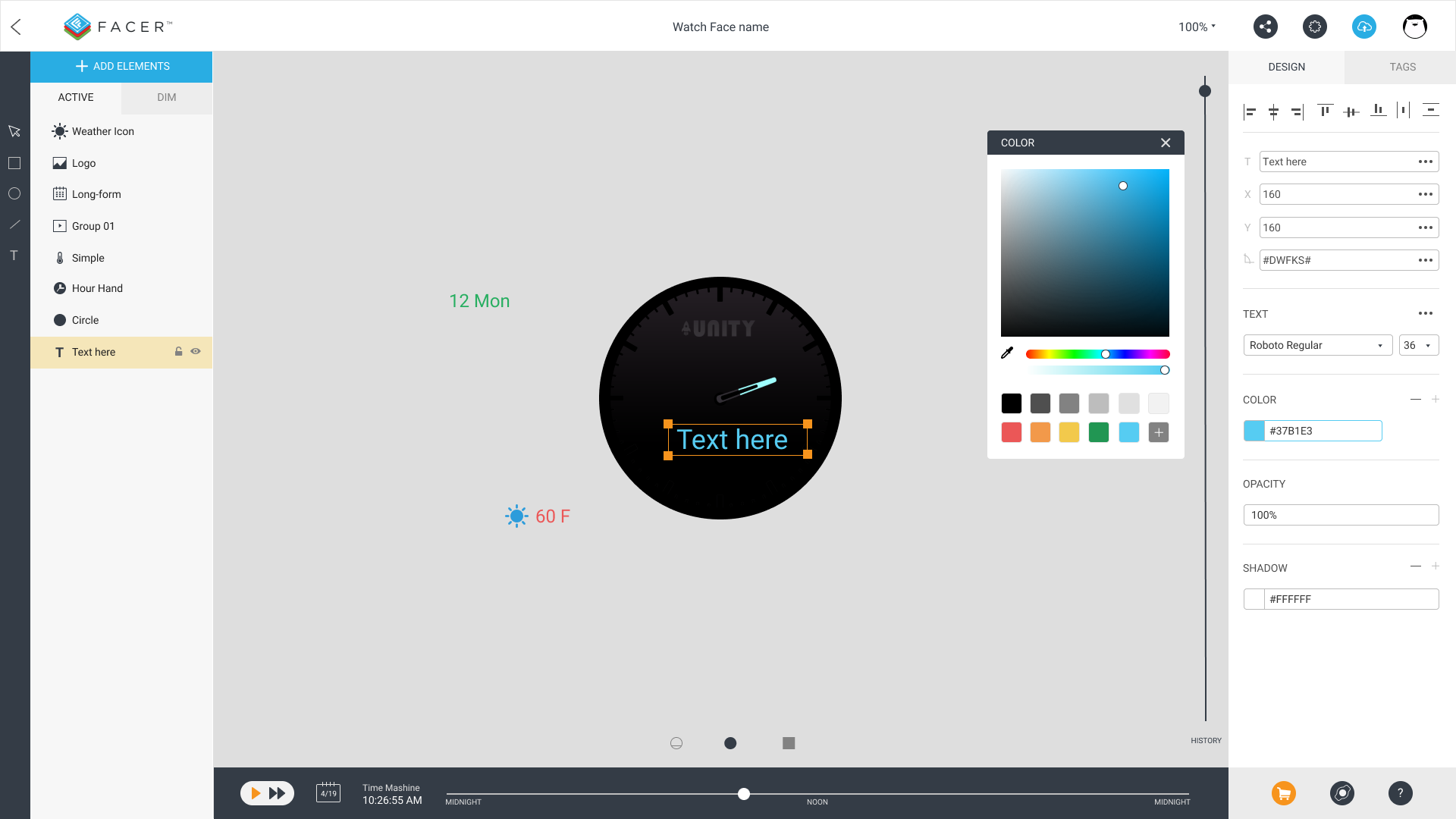1. Color picker looks more useful and can save a color to the library.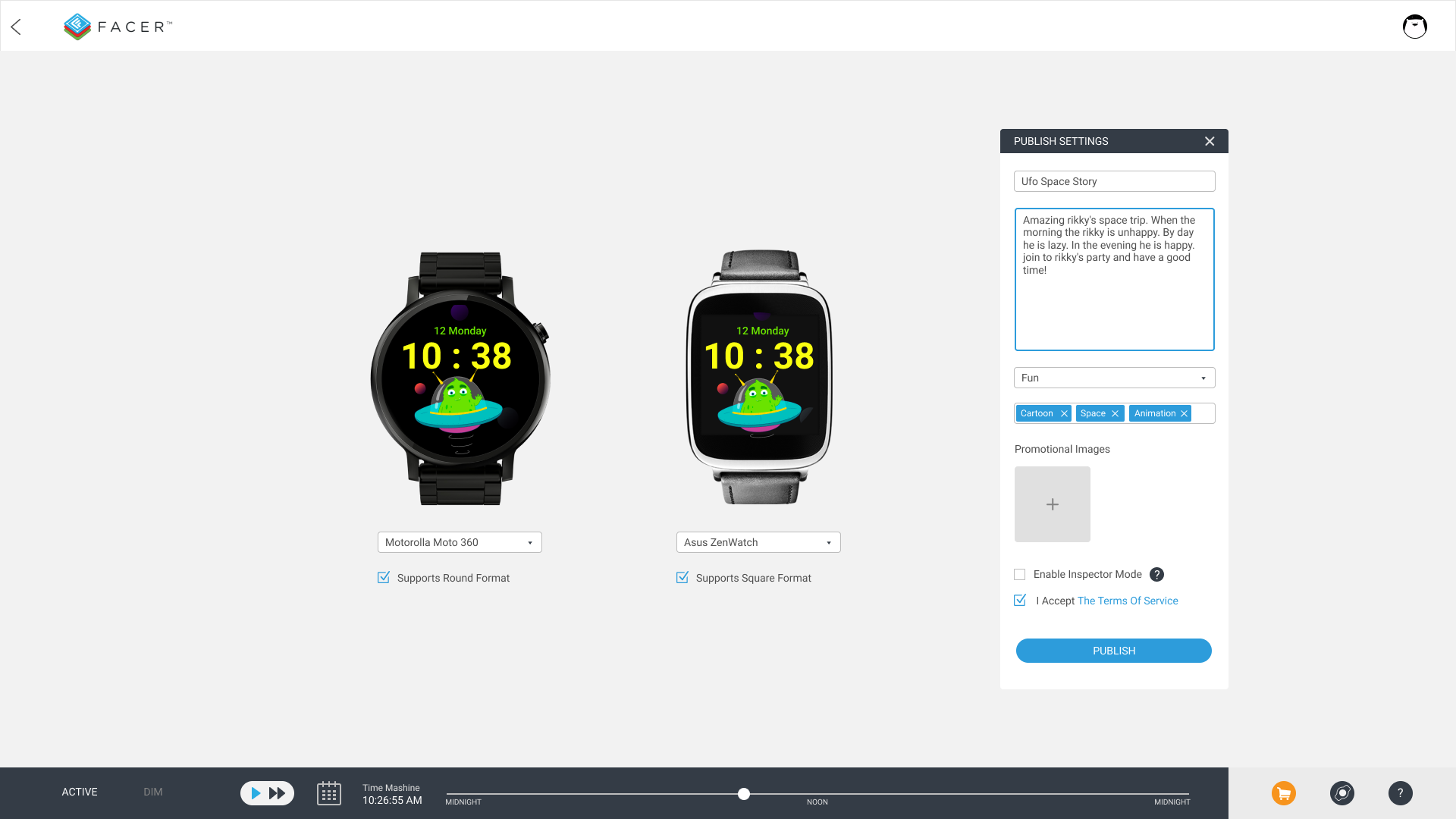1. Publish settings. New visualisation.

If something wrong with images loading go to original article on my site
http://faustland.ru/work/facer_demo.html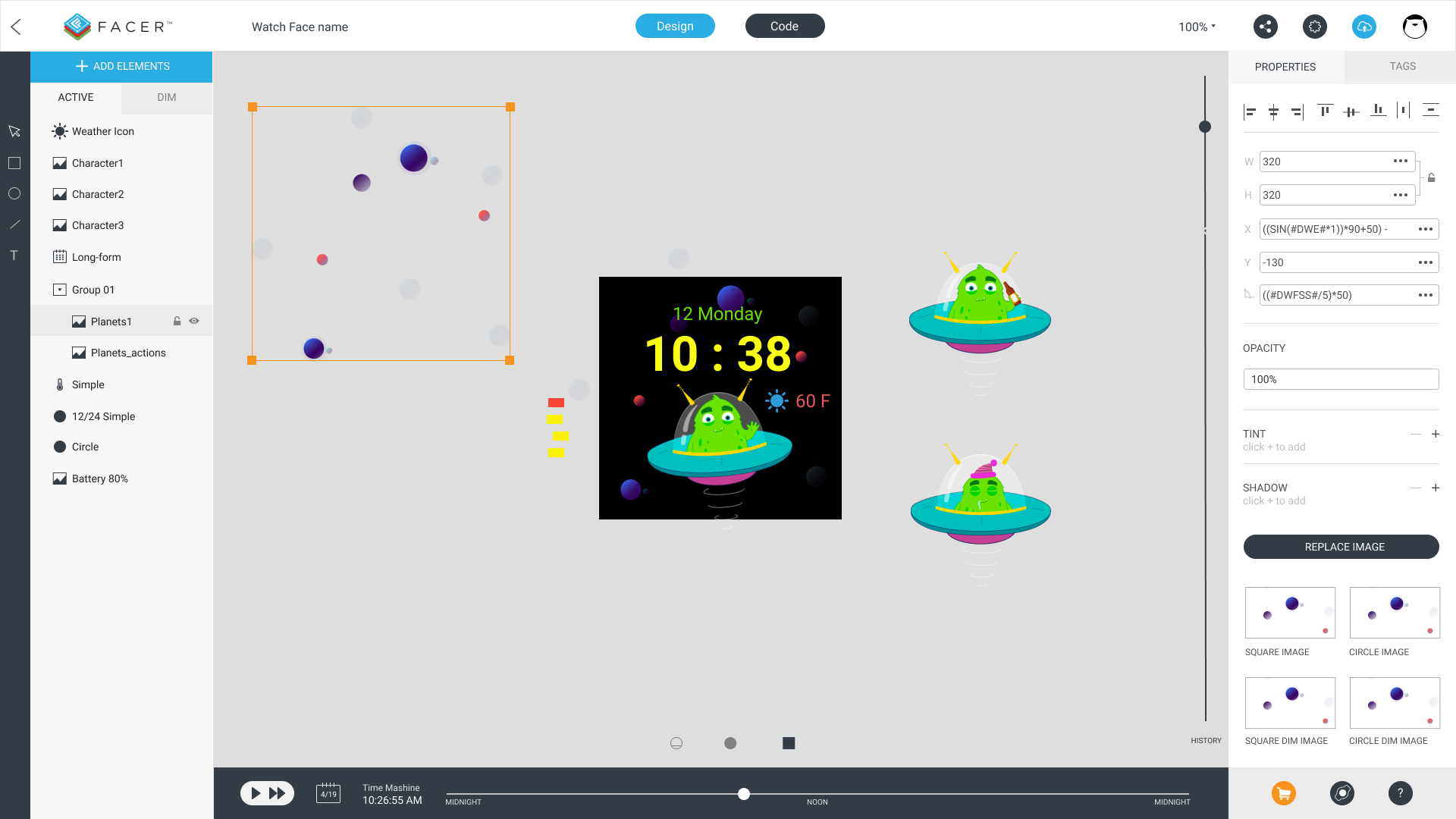So, I addad some new features in my mockups. Look at top bar. There are to modes. Designe mode and Code mode.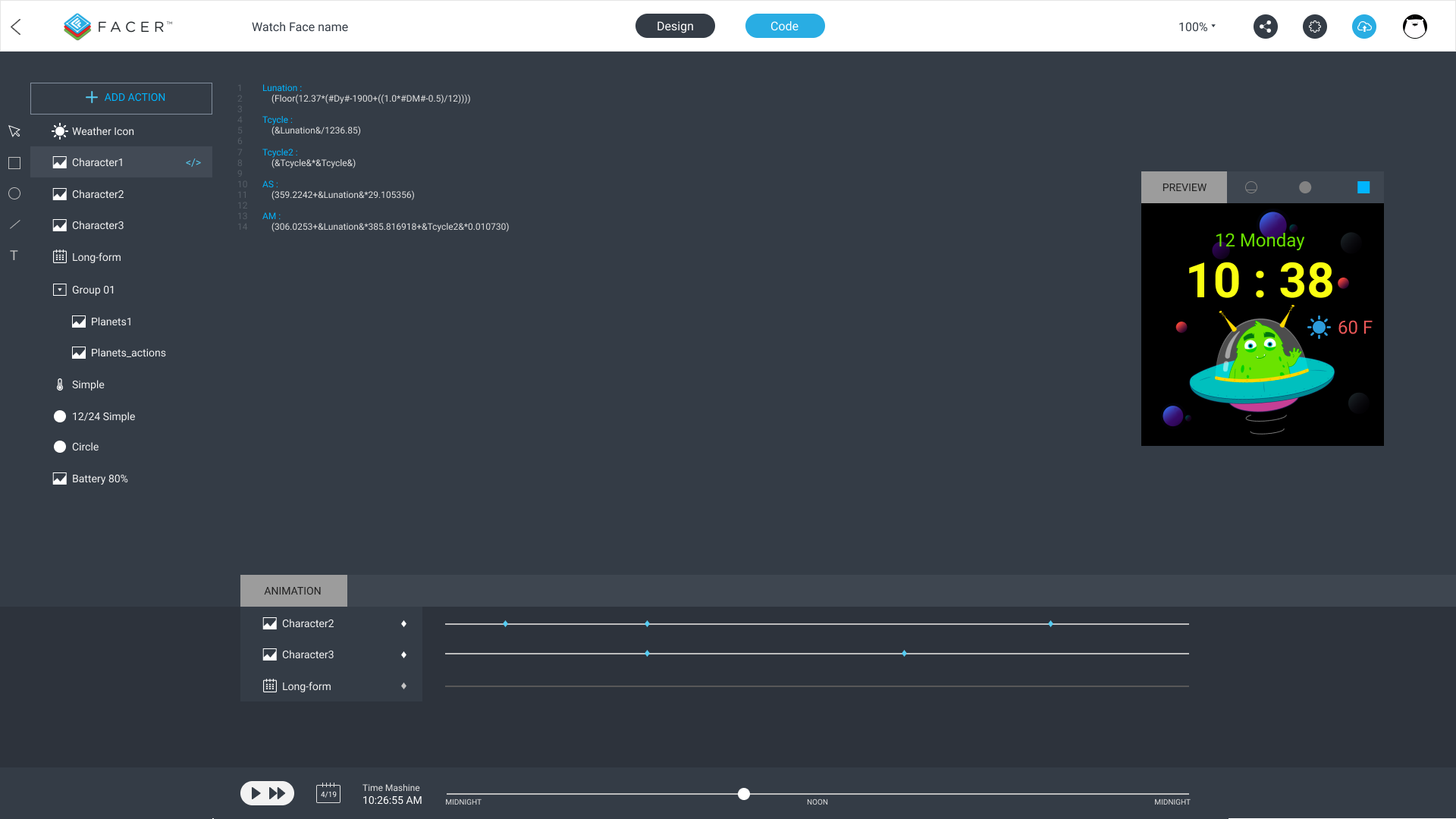In the Code mode you can see which layers are coding now, preview window and animation time machine. This is only concept. Animation works like in Adobe After Effects.

Wow! Great UI!

2 Likes

This is amazing @faustland! Great job!

All - are there specific parts of this suggested new UI that you’d like us to work on as a priority?

1 Like

Thanks. May be the priority things it’s possibility to multyply elements selection, group, visibility on canvas and more accurate/easy work with selection.

4 Likes

Really cool. I’ve been thinking about this for a while and have some great ideas as well. Just never put them to paper.

1 Like

I’d like to see more options in the standalone beta creator. The web version can stay the dumbed down one, but a more advanced version could be available as a desktop app.

1 Like

I see a lot of potentials in the ideas here.

Trust the users of the program, trust the developers of the program.

They know best, what is needed… and where it could lead to in future…

I think a lot of companies wish to have such creative, active and innovative human ressources potential…

Here are visionaires…

I at not as experienced as some of you guys but the frustrations of not being able to zoom or have folders I am sure goes from new people to experienced ones. I guess I am used to Illustrator. This UI is great without feeling like I am being bombarded with too much. I agree about the web portal simple or at least a way to turn on beginner or advance mode. Since I will never be able to reach Pro level, hopefully these features won’t just be for them. Can we get some text on a curve love if possible? Another thought coming from a math newbie, maybe animated idea of what the math does. There are some great tutorials but seeing the what the code does helps to know what I am looking for, or at least a link to the well written tutorials. When you don’t know what you are supposed to put in the search bar can steer some new people away.
Facer, this user has something worth doing.

IT SEEMS ME A GREAT JOB!

I think the priority would be to group layers and block them!
in my case it would be great since I work with an immense amount of layers!

3 Likes

Yes. Grouping of elements/layers was my wish as well. Some of my watch faces have about 50 layers.

5 Likes

Grouping of layers would be great as well as a zoom. I think the stand alone creator should have an option to save to hard drive and then sync to facer servers later. This way you can create offline if your not in an area with internet.

3 Likes

more intuitive interface, I like it

How about grouping of layers? Did someone mention grouping of layers yet? I’d like some grouping of layers. Let’s group layers.

I’d actually like some variables too. With programming being a hobby, I’m used to setting variables so that I can use them in different places without having to update in multiple places if I change them. It would be nice if I use a duplicate of a formula to able to write it once in one place and then only update there.

Example:
\$(#DWFSS#-(60*(floor(#Ds#/10))))>=55?((60*(floor(#Ds#/10)))-660)+((#DWFSS#-(60*(floor(#Ds#/10))))12):(60(floor(#Ds#/10)))\$

Variable @T@

``````(60*(floor(#Ds#/10)))
``````

Variable @M@

``````60
``````

Variable @C@

``````55
``````

Variable @S@

``````(@M@/(@M@-@C@))
``````

Variable @O@

``````@S@*@C@
``````

Actual formula

``````\$(#DWFSS#-@T@)>=@C@?(@T@-@O@)+((#DWFSS#-@T@)*@S@):@T@\$
``````

Now I only need to change one value at one place to get the rest of the values I need without having to calculate and update it manually. This would allow for some pretty complex formulas that would still be simple to follow and change.

1 Like

@faustland

I’ve been thinking about this lately due to the difficulty in testing. I wish there was a Master Testing Page. Here are some of the ideas:

Accelerometer Tester: something to move the watch face in each direction. You could move the box or center point on a circle and also have boxes to enter a specific coordinate.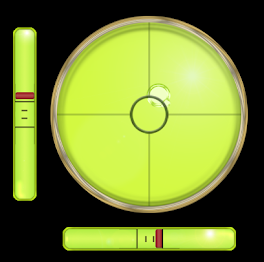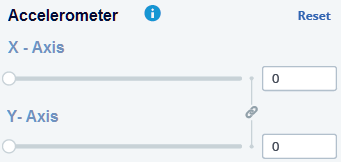Heart Rate/Step Count/Temp: A simple player style UI. You could enter a min and max, then “play” the heart rate and see it increase over time. You could also enter a static number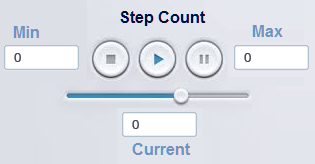Some other things that could be tested:

• Random Functions
• Weather Condition
2 Likes

one other thought. I would add the ability to increase the size of the canvas, also add in a ruler and the ability to drag down guide lines:

3 Likes

More ideas:

More Text Options

• Vertical type
• Warp
2 Likes

I completely agree about canvas size, it can be frustrating when I want to zoom in and can’t. I agree on guidelines/rulers to help with placement. Especially when Facer resizes (wish I knew what ratio it does so). Thank you for backing up my suggestion of more type options. Did anyone mention Clipping Masks?

1 Like

`(((((floor(365.25*(#DM#<2?(#Dy#-#Dy#):#Dy#)))+(floor(30.6001*((#DM#<=2)?((#DM#+1)+12):(#DM#+1))))+#Dd#+1720995)+2-((#Dd#+31*(#DM#+12*#Dy#))>=(15+31*(10+12*1582))?(floor(0.01*((#DM#<=2)?(#Dy#-#Dy#):(#Dy#)))):(#DM#<=2?(#Dy#-#Dy#):(#Dy#)))+floor(0.25*((#Dd#+31*(#DM#+12*#Dy#))>=(15+31*(10+12*1582))?(floor(0.01*((#DM#<=2)?(#Dy#-#Dy#):(#Dy#)))):(#DM#<=2?(#Dy#-#Dy#):(#Dy#)))))-((2415020+28*((floor(12.37*(#Dy#-1900+((1.0*#DM#-0.5)/12.0))))))+((0.75933+1.53058868*((floor(12.37*(#Dy#-1900+((1.0*#DM#-0.5)/12.0)))))+((1.178e-4)-(1.55e-7)*(((floor(12.37*(#Dy#-1900+((1.0*#DM#-0.5)/12.0)))))/1236.85))*((((floor(12.37*(#Dy#-1900+((1.0*#DM#-0.5)/12.0)))))/1236.85)*(((floor(12.37*(#Dy#-1900+((1.0*#DM#-0.5)/12.0)))))/1236.85))+(0.1734-3.93e-4*(((floor(12.37*(#Dy#-1900+((1.0*#DM#-0.5)/12.0)))))/1236.85))*Math.sin(0.017453292500000002*(359.2242+29.105356*((floor(12.37*(#Dy#-1900+((1.0*#DM#-0.5)/12.0)))))))-0.4068*Math.sin(0.017453292500000002*(306.0253+385.816918*((floor(12.37*(#Dy#-1900+((1.0*#DM#-0.5)/12.0)))))+0.010730*((((floor(12.37*(#Dy#-1900+((1.0*#DM#-0.5)/12.0)))))/1236.85)*(((floor(12.37*(#Dy#-1900+((1.0*#DM#-0.5)/12.0)))))/1236.85)))))>0.0?(floor((0.75933+1.53058868*((floor(12.37*(#Dy#-1900+((1.0*#DM#-0.5)/12.0)))))+((1.178e-4)-(1.55e-7)*(((floor(12.37*(#Dy#-1900+((1.0*#DM#-0.5)/12.0)))))/1236.85))*((((floor(12.37*(#Dy#-1900+((1.0*#DM#-0.5)/12.0)))))/1236.85)*(((floor(12.37*(#Dy#-1900+((1.0*#DM#-0.5)/12.0)))))/1236.85))+(0.1734-3.93e-4*(((floor(12.37*(#Dy#-1900+((1.0*#DM#-0.5)/12.0)))))/1236.85))*Math.sin(0.017453292500000002*(359.2242+29.105356*((floor(12.37*(#Dy#-1900+((1.0*#DM#-0.5)/12.0)))))))-0.4068*Math.sin(0.017453292500000002*(306.0253+385.816918*((floor(12.37*(#Dy#-1900+((1.0*#DM#-0.5)/12.0)))))+0.010730*((((floor(12.37*(#Dy#-1900+((1.0*#DM#-0.5)/12.0)))))/1236.85)*(((floor(12.37*(#Dy#-1900+((1.0*#DM#-0.5)/12.0)))))/1236.85))))))):(ceil((0.75933+1.53058868*((floor(12.37*(#Dy#-1900+((1.0*#DM#-0.5)/12.0)))))+((1.178e-4)-(1.55e-7)*(((floor(12.37*(#Dy#-1900+((1.0*#DM#-0.5)/12.0)))))/1236.85))*((((floor(12.37*(#Dy#-1900+((1.0*#DM#-0.5)/12.0)))))/1236.85)*(((floor(12.37*(#Dy#-1900+((1.0*#DM#-0.5)/12.0)))))/1236.85))+(0.1734-3.93e-4*(((floor(12.37*(#Dy#-1900+((1.0*#DM#-0.5)/12.0)))))/1236.85))*Math.sin(0.017453292500000002*(359.2242+29.105356*((floor(12.37*(#Dy#-1900+((1.0*#DM#-0.5)/12.0)))))))-0.4068*Math.sin(0.017453292500000002*(306.0253+385.816918*((floor(12.37*(#Dy#-1900+((1.0*#DM#-0.5)/12.0)))))+0.010730*((((floor(12.37*(#Dy#-1900+((1.0*#DM#-0.5)/12.0)))))/1236.85)*(((floor(12.37*(#Dy#-1900+((1.0*#DM#-0.5)/12.0)))))/1236.85)))))-1.0))))+30)%30)`